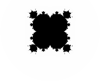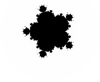# Visual C# 2005 (Windows) GuideFractals: Mandelbrot Variations

Remember that all of our code so far is based on that simple formula,

z → z2 + c

Different powers of z in this formula, produce other sets that are similar in nature to the Mandelbrot set. They are often referred to as Multibrot sets.z → z3 + c z4 + c z → z5 + c z → z6 + c

You can see that the number of 'bulbs' in the image increases as the power of z increases.

To draw a multibrot set, you need a small variation in the IsInMandelbrotSet() procedure. It is basically a change in the way that the new values of a and b are calculated. These changes are,

### z → z3 + c

```a = a * (aSquare - (3 * bSquare)) + startA; b = b * ((3 * aSquare) - bSquare) + startB;```

### z → z4 + c

```newA = (aSquare * aSquare) + (bSquare * bSquare) - (6 * aSquare * bSquare) + startA; newB = ((4 * a * b) * (aSquare - bSquare)) + startB; a = newA; b = newB;```

### z → z5 + c

```newA = (a * aSquare * aSquare) - (10 * a * aSquare * bSquare) + (5 * a * bSquare * bSquare) + startA; newB = (5 * b * aSquare * aSquare) - (10 * aSquare * b * bSquare) + (bSquare * bSquare * b) + startB; a = newA; b = newB;```

### z → z6 + c

```newA = (aSquare * aSquare * aSquare) - (15 * aSquare * aSquare * bSquare) + (15 * aSquare * bSquare * bSquare) - (bSquare * bSquare * bSquare) + startA; newB = (6 * aSquare * aSquare * a * b) - (20 * aSquare * a * bSquare * b) + (6 * a * bSquare * bSquare * b) + startB; a = newA; b = newB;```

The procedures get a bit beastly after a while.

There are also plottable images for negative powers of z too.